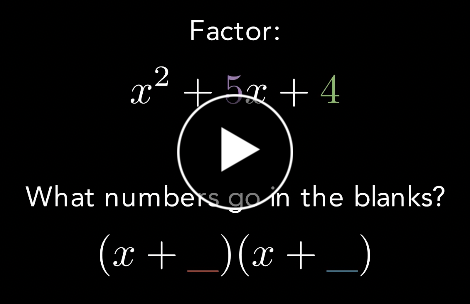# Factoring Calculator

#### What do you want to calculate?

Example: x^2+5x+4

### How to factor expressions

If you are factoring a quadratic like x^2+5x+4 you want to find two numbers that
• Add up to 5
• Multiply together to get 4
Since 1 and 4 add up to 5 and multiply together to get 4, we can factor it like:
(x+1)(x+4)

### Current calculator limitations

• Doesn't support multivariable expressions
• If you have an expression that you want the calculator to support in the future, please contact us

### Factoring Expressions Video LessonHow To Factor x^2+5x+4 [0:58]

Need more problem types? Try MathPapa Algebra Calculator